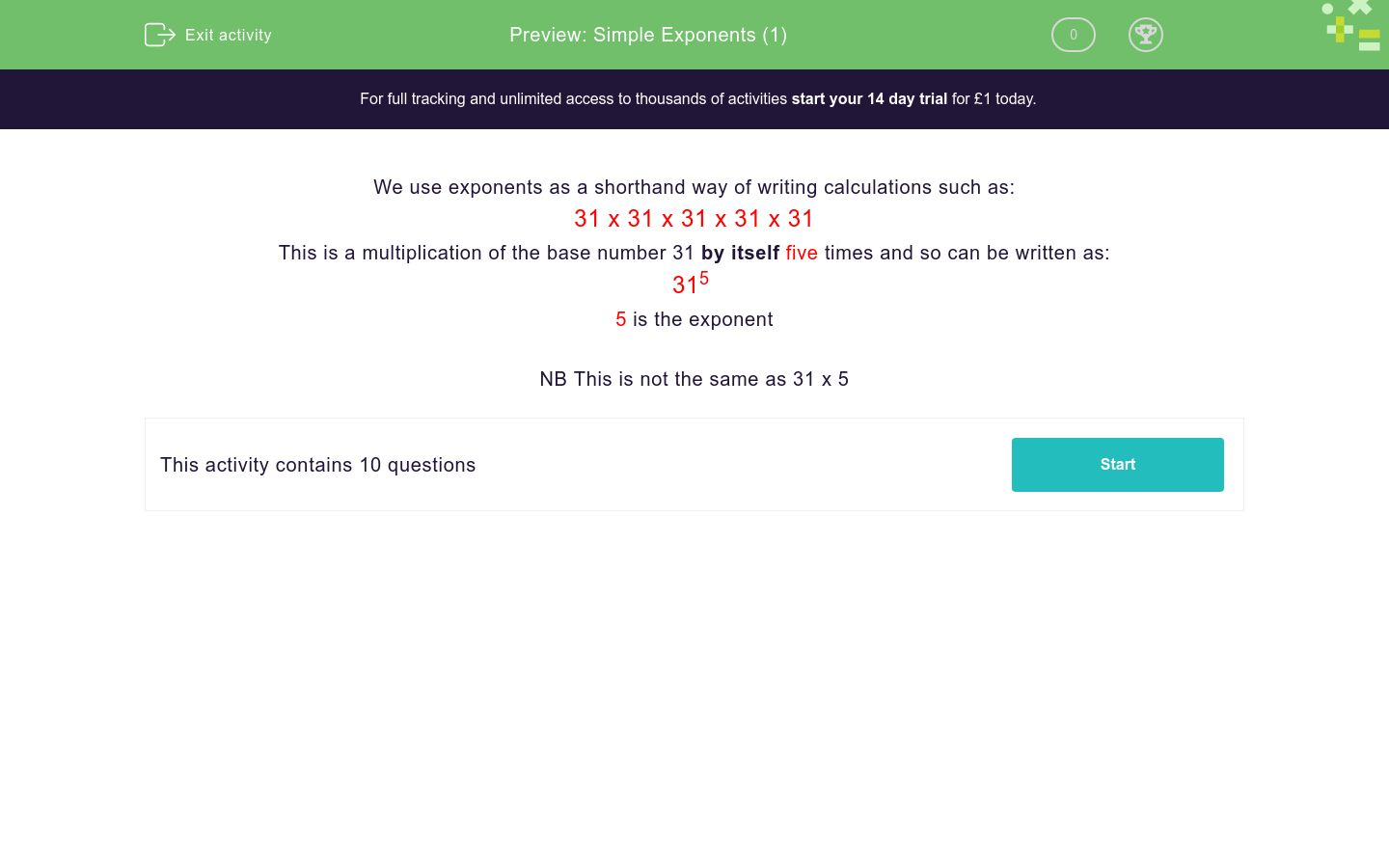# Simple Exponents (1)

In this worksheet, students understand and use simple exponents.Key stage:  KS 3

Curriculum topic:   Number

Curriculum subtopic:   Understand Integer Powers/Real Roots

Difficulty level:### QUESTION 1 of 10

We use exponents as a shorthand way of writing calculations such as:

31 x 31 x 31 x 31 x 31

This is a multiplication of the base number 31 by itself five times and so can be written as:

315

5 is the exponent

NB This is not the same as 31 x 5

What is the exponent n when we write the following as 31n: (just write the exponent)

31 x 31 x 31 x 31

What is the exponent n when we write the following as 31n: (just write the exponent)

31 x 31 x 31 x 31 x 31 x 31 x 31

What is the exponent n when we write the following as 31n: (just write the exponent)

31 x 31 x 31

What is the exponent n when we write the following as 3n: (just write the exponent)

3 x 3

What is the exponent n when we write the following as 3n: (just write the exponent)

3 x 3 x 3 x 3 x 3 x 3 x 3 x 3

What is the exponent n when we write the following as 3n: (just write the exponent)

3 x 3 x 3 x 3 x 3 x 3 x 3 x 3 x 3 x 3

What is the exponent n when we write the following as 23n: (just write the exponent)

23 x 23 x 23 x 23 x 23

What is the exponent n when we write the following as 23n: (just write the exponent)

23 x 23 x 23 x 23 x 23 x 23 x 23

What is the exponent n when we write the following as 23n: (just write the exponent)

23

What is the exponent n when we write the following as 203n: (just write the exponent)

203 x 203 x 203

• Question 1

What is the exponent n when we write the following as 31n: (just write the exponent)

31 x 31 x 31 x 31

4
• Question 2

What is the exponent n when we write the following as 31n: (just write the exponent)

31 x 31 x 31 x 31 x 31 x 31 x 31

7
• Question 3

What is the exponent n when we write the following as 31n: (just write the exponent)

31 x 31 x 31

3
• Question 4

What is the exponent n when we write the following as 3n: (just write the exponent)

3 x 3

2
• Question 5

What is the exponent n when we write the following as 3n: (just write the exponent)

3 x 3 x 3 x 3 x 3 x 3 x 3 x 3

8
• Question 6

What is the exponent n when we write the following as 3n: (just write the exponent)

3 x 3 x 3 x 3 x 3 x 3 x 3 x 3 x 3 x 3

10
• Question 7

What is the exponent n when we write the following as 23n: (just write the exponent)

23 x 23 x 23 x 23 x 23

5
• Question 8

What is the exponent n when we write the following as 23n: (just write the exponent)

23 x 23 x 23 x 23 x 23 x 23 x 23

7
• Question 9

What is the exponent n when we write the following as 23n: (just write the exponent)

23

1
• Question 10

What is the exponent n when we write the following as 203n: (just write the exponent)

203 x 203 x 203

3
---- OR ----

Sign up for a £1 trial so you can track and measure your child's progress on this activity.

### What is EdPlace?

We're your National Curriculum aligned online education content provider helping each child succeed in English, maths and science from year 1 to GCSE. With an EdPlace account you’ll be able to track and measure progress, helping each child achieve their best. We build confidence and attainment by personalising each child’s learning at a level that suits them.

Get started